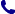• Kontakt
• Newsletter Zapisz się po informacje o nowościach i specjalnych ofertach. Administrator danych: ABE-IPS sp. z o.o.
Wybierz interesujące Cię kategorie
Wyrażam zgodę na przetwarzanie moich danych osobowych w celach marketingowych, tym samym akceptuję Regulamin Newslettera i Politykę prywatności.
Wyrażam zgodę na przesyłanie informacji handlowych drogą elektroniczną.
Wypisz się
• Klienci instytucjonalni
• Dostawa
• O nas
Dostęp on-line

# Książki

0.00 PLN
Schowek (0)
 Schowek jest pusty

## Statistics in Kinesiology

 Autorzy Wydawnictwo Human Kinetics Publishers Data wydania 16/03/2020 Wydanie Piąte Liczba stron 312 Forma publikacji książka w miękkiej oprawie, dzieło wielotomowe Liczba tomów 1 Poziom zaawansowania Dla szkół wyższych i kształcenia podyplomowego ISBN 9781492560715 Kategorie Epidemiologia i statystyka medyczna, Biomechanika, kinetyka człowieka, Prawdopodobieństwo i statystyka Zastępuje 9781450402545
328.86 PLN (z VAT)
\$89.45 / €73.33 / £63.00 /
Produkt na zamówienie
Dostawa 3-4 tygodnie
Ilość
Do schowka

## Opis książki

Textbook undergraduate courses in statistics or measurement in kinesiology and exercise science programs; a reference for professionals in movement sciences.

Statistics in Kinesiology

## Spis treści

Chapter 1. Measurement, Statistics, and Research
What Is Measurement?
Process of Measurement
Variables and Constants
Research Design and Statistical Analysis
Statistical Inference
Summary

Chapter 2. Organizing and Displaying Data
Organizing Data
Displaying Data
Summary

Chapter 3. Percentiles
Common Percentile Divisions
Calculations Using Percentiles
Summary

Chapter 4. Measures of Central Tendency
Mode
Median
Mean
Relationships Among the Mode, Median, and Mean
Summary

Chapter 5. Measures of Variability
Range
Interquartile Range
Variance
Standard Deviation
Definition Method of Hand Calculations
Calculating Standard Deviation for a Sample
Coefficient of Variation
Standard Deviation and Normal Distribution
Summary

Chapter 6. The Normal Curve
Z Scores
Standard Scores
Probability and Odds
Calculating Skewness and Kurtosis
Summary

Chapter 7. Fundamentals of Statistical Inference
Predicting Population Parameters Using Statistical Inference
Estimating Sampling Error
Levels of Confidence, Confidence Intervals, and Probability of Error
An Example Using Statistical Inference
Statistical Hypothesis Testing
Type I and Type II Error
Degrees of Freedom
Living With Uncertainty
Two- and One-Tailed Tests
Applying Confidence Intervals
Summary

Chapter 8. Correlation and Bivariate Regression
Correlation
Calculating the Correlation Coefficient
Bivariate Regression
Homoscedasticity
Summary

Chapter 9. Multiple Correlation and Multiple Regression
Multiple Correlation
Partial Correlation
Multiple Regression
Summary

Chapter 10. The t Test: Comparing Means From Two Sets of Data
The t Tests
Types of t Tests
Magnitude of the Difference (Size of Effect)
Determining Power and Sample Size
The t Test for Proportions
Summary

Chapter 11. Simple Analysis of Variance: Comparing the Means Among Three or More Sets of Data
Assumptions in ANOVA
Sources of Variance
Calculating F: The Definition Method
Determining the Significance of F
Post Hoc Tests
Magnitude of the Treatment (Size of Effect)
Summary

Chapter 12. Analysis of Variance With Repeated Measures
Assumptions in Repeated Measures ANOVA
Calculating Repeated Measures ANOVA
Correcting for Violations of the Assumption of Sphericity
Post Hoc Tests
Interpreting the Results
An Example From Leisure Studies and Recreation
Summary

Chapter 13. Quantifying Reliability
Intraclass Correlation Coefficient
Standard Error of Measurement
Summary

Chapter 14. Factorial Analysis of Variance
A Between-Between Example
A Between-Within Example
A Within-Within Example
Summary

Chapter 15. Analysis of Covariance
Relationship Between ANOVA and Regression
ANCOVA and Statistical Power
Assumptions in ANCOVA
The Pretest-Posttest Control Group Design
Pairwise Comparisons
Summary

Chapter 16. Analysis of Nonparametric Data
Chi-Square (Single Classification)
Chi-Square (Two or More Classifications)
Rank Order Correlation
Mann-Whitney U Test
Kruskal-Wallis ANOVA for Ranked Data
Friedman's Two-Way ANOVA by Ranks
Summary

Chapter 17. Clinical Measures of Association
Relative Risk
Odds Ratio
Diagnostic Testing
Summary801 777 223